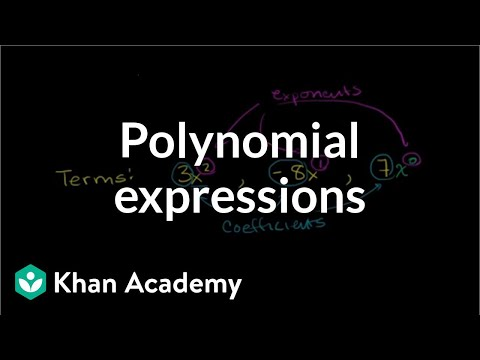### Algebra: Polynomials

115 votes
Free Closed [?]Using polynomial expressions and factoring polynomials.
Terms coefficients and exponents in a polynomial. Interesting Polynomial Coefficient Problem. Polynomials1. Polynomials 2. Evaluating a polynomial at a given value. Simplify a polynomial. Adding Polynomials. Example: Adding polynomials with multiple variables. Addition and Subtraction of Polynomials. Adding and Subtracting Polynomials 1. Adding and Subtracting Polynomials 2. Adding and Subtracting Polynomials 3. Subtracting Polynomials. Subtracting polynomials with multiple variables. Adding and subtracting polynomials. Multiplying Monomials. Dividing Monomials. Multiplying and Dividing Monomials 1. Multiplying and Dividing Monomials 2. Multiplying and Dividing Monomials 3. Monomial Greatest Common Factor. Factoring and the Distributive Property. Factoring and the Distributive Property 2. Factoring and the Distributive Property 3. Multiplying Binomials with Radicals. Multiplication of Polynomials. Multiplying Binomials. Multiplying Polynomials1. Multiplying Polynomials 2. Square a Binomial. Special Products of Binomials. Special Polynomials Products 1. Factor polynomials using the GCF. Special Products of Polynomials 1. Special Products of Polynomials 2. Multiplying expressions 0.5. Factoring linear binomials. Multiplying expressions 1. Multiplying Monomials by Polynomials. Multiplying Polynomials. Multiplying Polynomials 3. More multiplying polynomials. Multiplying polynomials. Level 1 multiplying expressions. Polynomial Division. Polynomial divided by monomial. Dividing multivariable polynomial with monomial. Dividing polynomials 1. Dividing polynomials with remainders. Synthetic Division. Synthetic Division Example 2. Why Synthetic Division Works. Factoring Sum of Cubes. Difference of Cubes Factoring. Algebraic Long Division. Algebra II: Simplifying Polynomials. Terms coefficients and exponents in a polynomial. Interesting Polynomial Coefficient Problem. Polynomials1. Polynomials 2. Evaluating a polynomial at a given value. Simplify a polynomial. Adding Polynomials. Example: Adding polynomials with multiple variables. Addition and Subtraction of Polynomials. Adding and Subtracting Polynomials 1. Adding and Subtracting Polynomials 2. Adding and Subtracting Polynomials 3. Subtracting Polynomials. Subtracting polynomials with multiple variables. Adding and subtracting polynomials. Multiplying Monomials. Dividing Monomials. Multiplying and Dividing Monomials 1. Multiplying and Dividing Monomials 2. Multiplying and Dividing Monomials 3. Monomial Greatest Common Factor. Factoring and the Distributive Property. Factoring and the Distributive Property 2. Factoring and the Distributive Property 3. Multiplying Binomials with Radicals. Multiplication of Polynomials. Multiplying Binomials. Multiplying Polynomials1. Multiplying Polynomials 2. Square a Binomial. Special Products of Binomials. Special Polynomials Products 1. Factor polynomials using the GCF. Special Products of Polynomials 1. Special Products of Polynomials 2. Multiplying expressions 0.5. Factoring linear binomials. Multiplying expressions 1. Multiplying Monomials by Polynomials. Multiplying Polynomials. Multiplying Polynomials 3. More multiplying polynomials. Multiplying polynomials. Level 1 multiplying expressions. Polynomial Division. Polynomial divided by monomial. Dividing multivariable polynomial with monomial. Dividing polynomials 1. Dividing polynomials with remainders. Synthetic Division. Synthetic Division Example 2. Why Synthetic Division Works. Factoring Sum of Cubes. Difference of Cubes Factoring. Algebraic Long Division. Algebra II: Simplifying Polynomials.

Categories:

# Reviews

-- no reviews yet -- make the first review

# Alternatives All alternatives to all Khan Academy courses

-- no alternatives found for the course --
If you know any alternatives, please let us know.

# Prerequisites

-- no prerequsites found for the course --
If you can suggest any prerequisite, please let us know.

# Paths

No Paths inclusing the course. You can build and share a path with this course included.

# Certification Exams

-- there are no exams to get certification after this course --
If your company does certification for those who completed this course then register your company as certification vendor and add your exams to the Exams Directory.

# Similar courses

Courses related to the course subject

### Advanced Algebraic Concepts and Applications in Mathematics

This course is ideal for people who want to gain a thorough understanding and knowledge of advanced topics in algebra. The advanc…

### Algebra

The foundations of Algebra from The Khan Academy.

### Algebra - Functions, Expressions and Equations

This free online course in algebra from ALISON will guide you through among other things expressions, systems of equations, functi…

### Algebra I

This undergraduate level Algebra I course covers groups, vector spaces, linear transformations, symmetry groups, bilinear forms, a…

### Algebra II

This undergraduate level course follows Algebra I. Topics include group representations, rings, ideals, fields, polynomial rings,…

### Algebra in Mathematics

This free online course offers a comprehensive introduction to algebra and carefully explains the concepts of algebraic fractions.

### Algebra: Absolute value

Understanding absolute value and solving absolute value equations and inequalities. Absolute Value and Number Lines. Absolute Valu…

### Algebra: ck12.org Algebra 1 Examples

Select problems from ck12.org's Algebra 1 FlexBook (Open Source Textbook). This is a good playlist to review if you want to make…

### Algebra: Conic sections

Identifying and graphing circles, ellipses, parabolas, and hyperbolas. Introduction to Conic Sections. Recognizing conic sections.

### Algebra: Exponent expressions and equations

Solving exponential and radical expressions and equations. Using scientific notation and significant figures. Negative and Positiv…

Let us know when you did the course Algebra: Polynomials.

 Started on: Completed on: Your grade (if any):Comments:Add the course Algebra: Polynomials to My Personal Education Path.

 Start the course on: Duration of study: 1 Week 2 Weeks 3 Weeks 4 Weeks 5 Weeks 6 Weeks 7 Weeks 8 Weeks 10 Weeks 12 Weeks 16 Weeks Notes:Successfully added to your path.Select what exam to connect to the course. The course will be displayed on the exam page in the list of courses supported for certification with the exam.

Notes about how the exam certifies students of the course (optional):